Next: Fine tuning a PID Up: number7 Previous: Improving the Controller

# The PID controller

We can use all three terms together and get the proportional-integral-derivative controller. The equation that fully describes the PID control is: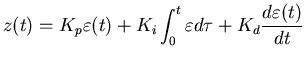where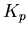is the proportional gain,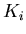is the integral gain and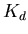is the differential gain. For a practical implementation on a digital system, the above equation is replaced with a discrete time approximation: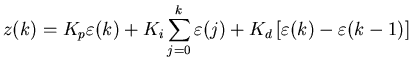where we have used a rectangle approximation to the integral and a backward difference approximation for the derivative. These are rather crude numerical approximations but we will generally be able to compensate for this by making the discrete sampling rate fast and by tuning the controller. The simple numerics has the great virtue of being very easy to implement.

Skip Carter 2008-08-20